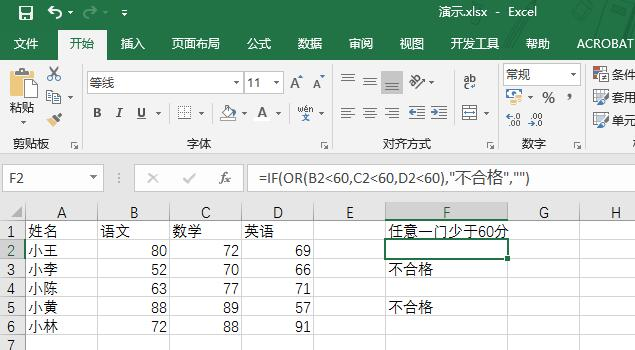# excel最基本的几种判断公式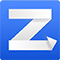1、借方显示正数，贷方显示负数
=IF(A2=0,-B2,A2)
A2  B2单元格为数字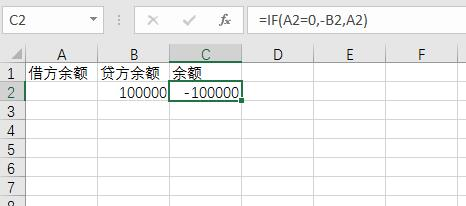2、错误值显示为空白
=IFERROR((C2-B2)/B2,"")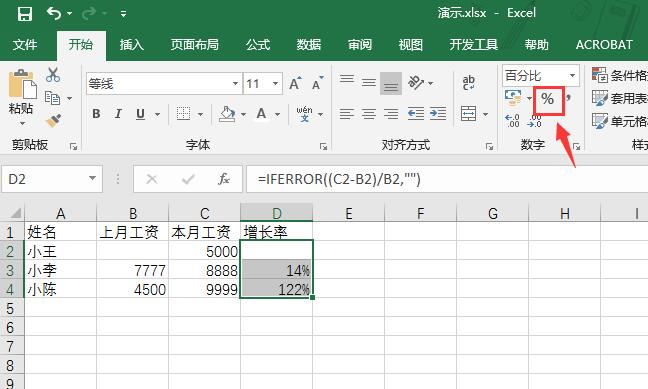PS：也可以根据需要，错误值显示为0或1
=IFERROR((C2-B2)/B2,1)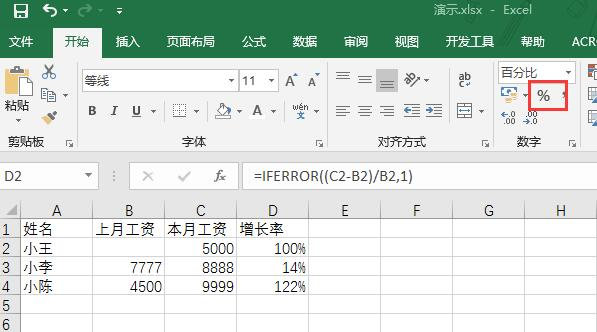3、IF多条件判断
=IF((B2>=70)*(C2>=70)*(D2>=70),A2,"")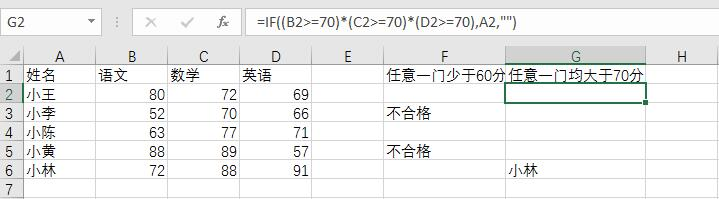PS:任何一个条件成立  IF+OR函数
=IF(OR(B2<60,C2<60,D2<60),"不合格","")